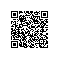# 一、简单贪心

Given n non-negative integers a1 , a2 , ..., an , where each represents a point at coordinate (i, ai ). n vertical lines are drawn such that the two endpoints of line i is at (i, ai ) and (i, 0). Find two lines, which together with x-axis forms a container, such that the container contains the most water.
Note: You may not slant the container.

• 这题在一分钟内就想了个笨方法，迅速敲完，KO！
• 因为题目还是很简单，不考虑将装水的容器倾斜，所以只要考虑梯形中最短的边和底边的乘积就行了：正反两遍循环数组，第一遍从0~len，先固定最左边的边，从右往左遍历数组，如果碰到大于等于最左边边长的边，那就判断是否大于当前的最大盛水体积并跟新，然后就停止这次循环，将最左边的边向右移动一格，重复操作；第二遍从len~0,和第一遍一样。

public class Solution {
public int maxArea(int[] height) {
int len=height.length;
if(len==0){
return 0;
}
int maxW=0;
for(int i=0;i<len;i++){
for(int j=len-1;j>i;j--){
if(height[j]>=height[i]){
if(height[i]*(j-i)>maxW){
maxW=height[i]*(j-i);
}
break;
}
}
}
for(int i=len-1;i>0;i--){
for(int j=0;j<i;j++){
if(height[j]>=height[i]){
if(height[i]*(i-j)>maxW){
maxW=height[i]*(i-j);
}
break;
}
}
}
return maxW;
}
}

• 好吧，虚心学习，看了一下其他人的代码。发现一个好方法：
• 从两边往中间走，每次舍弃较短的边，因为宽度减小，要通过高度的增加来弥补

public class Solution {
public int maxArea(int[] height) {
int len=height.length;
if(len==0){
return 0;
}
int l=0;
int r=len-1;
int maxW=0;
while(l<r){
maxW=Math.max(maxW,Math.min(height[l],height[r])*(r-l));
if(height[l]<height[r]){
l++;
}
else{
r--;
}
}
return maxW;
}
}

# 二、简单回文数字

Determine whether an integer is a palindrome. Do this without extra space.
click to show spoilers.
Some hints:
Could negative integers be palindromes? (ie, -1)
If you are thinking of converting the integer to string, note the restriction of using extra space.
You could also try reversing an integer. However, if you have solved the problem "Reverse Integer", you know that the reversed integer might overflow. How would you handle such case?
There is a more generic way of solving this problem.

• 题目有个限制，就是不能开辟新的空间。
• 那就和一般的回文串做法不同了，可以这么来搞：干脆求出它翻转过来的整型，最后比较是否值相等。
• 想到这个思路，是因为int型的特殊基本数据类型，特别对待，哈哈哈

public class Solution {
public boolean isPalindrome(int x) {
if(x<0){
return false;
}
int reverse=0;
int x_rel=x;
while(x!=0){
reverse=reverse*10+x%10;
x/=10;
}
return  reverse==x_rel;
}
}

# 三、字符串，模拟

Implement atoi to convert a string to an integer.
Hint: Carefully consider all possible input cases. If you want a challenge, please do not see below and ask yourself what are the possible input cases.
Notes: It is intended for this problem to be specified vaguely (ie, no given input specs). You are responsible to gather all the input requirements up front.
spoilers alert... click to show requirements for atoi.
Requirements for atoi:
The function first discards as many whitespace characters as necessary until the first non-whitespace character is found. Then, starting from this character, takes an optional initial plus or minus sign followed by as many numerical digits as possible, and interprets them as a numerical value.
The string can contain additional characters after those that form the integral number, which are ignored and have no effect on the behavior of this function.
If the first sequence of non-whitespace characters in str is not a valid integral number, or if no such sequence exists because either str is empty or it contains only whitespace characters, no conversion is performed.
If no valid conversion could be performed, a zero value is returned. If the correct value is out of the range of representable values, INT_MAX (2147483647) or INT_MIN (-2147483648) is returned.

• 细节要求比较多，正负号，非法字符，溢出等都要考虑。

public class Solution {
public int atoi(String str) {
if(str==null||str.length()==0){
return 0;
}
int pos=0;
while(str.charAt(pos)==' '){
pos++;
}
int flag=1;
if(str.charAt(pos)=='-'){
flag=-1;
pos++;
}
if(str.charAt(pos)=='+'){
flag=1;
pos++;
}
int len=str.length();
int res=0;
for(int i=pos;i<len;i++){
char c=str.charAt(i);
if(c<'0'||c>'9'){
break;
}
if(res>Integer.MAX_VALUE/10||(res==Integer.MAX_VALUE/10&&c>'7')){
if(flag>0){
return Integer.MAX_VALUE;
}
else{
return Integer.MIN_VALUE;
}
}
res=res*10+(c-'0');
}
return res*flag;
}
}使用钉钉扫一扫加入圈子
+ 订阅